CSE 5311 Fall 1999

Quiz #5

1.
Professor Midas drives an automobile between two cities m miles apart along an interstate highway. His car can go n miles on a tank of gas, and he starts with a full tank. His map indicates that there are g gas stations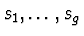along the way, and d(si) is the distance of station ifrom the previous station si-1. The distance to the first gas station is d(s1), and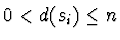for all i. The professor wishes to minimize the number of gas stops during the trip.

(a)
(8 points.) Prove that this road-trip problem exhibits optimal substructure.

Let the start city be s0, the destination city be d, and the optimal solution to the trip by the stops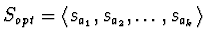. Let cost(S,c1,c2) be the cost of a solution S for traveling from point c1 to c2. Therefore, cost(Sopt,s0,d) = k.

Define a subproblem of the original problem to be the problem of traveling from the first stop sa1 in the solution to the destination city d. In terms of this subproblem the cost of the optimal solution can be written as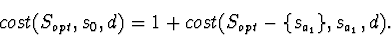Assume there is a better solution S' to the subproblem of traveling from sa1 to d, such that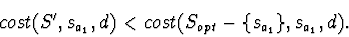However, if this better solution S' exists, then we can use it after our stop at sa1 to achieve a lower-cost solution to the original problem.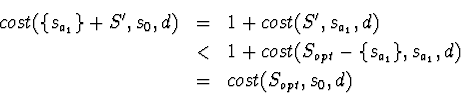This contradicts the optimality of Sopt, and therefore a better solution to the subproblem cannot exist. Thus, the optimal solution to the original problem contains optimal solutions to the subproblems, i.e., the road-trip problem exhibits optimal substructure.

(b)
(12 points.) Describe a greedy algorithm for solving the road-trip problem and prove that your algorithm yields an optimal solution.

The greedy algorithm that leads to an optimal solution is to always go to the farthest stop you can reach on a tank of gas until you reach the destination city.

Since we have already proven optimal substructure for this problem, to show our greedy choice leads to an optimal solution, we need only show that our greedy choice satisfies the greedy choice property, i.e., that the greedy choice is in an optimal solution and can be made first. To show that the greedy choice is in an optimal solution, we will show that an optimal solution not containing the greedy choice can be changed to include the greedy choice without sacrificing optimality (i.e., minimal number of stops).

For the top-level problem of traveling from s0 to d, let sg be the greedy choice for the first stop, and let sopt be the first stop specified by an optimal solution. First note that sopt must be either the same as or before sg. If sopt were after sg, then the greedy choice would choose sopt over sg. Also, if sopt = sg, then we have proven our hypothesis. Lastly, if sg is after sopt, then we can replace sopt by sg while maintaining the same minimal number of stops. Thus, the greedy choice is in an optimal solution. Also, since we have shown that the first greedy choice can be the first stop of an optimal solution, the greedy choice can be made first. Optimal substructure completes the inductive proof that our greedy choice leads to an optimal solution.